## A strange interface bug...

The procedure F computes the smallest prime factor of  ithprime(n)-2

F := n -> ifactors((ithprime(n)-2)):

F(11);
29
Strangely, print(F)  must be interrupted!
print(F);
Warning,  computation interrupted

Also,

F := n -> ifactors((ithprime(n)-2));

Warning,  computation interrupted
Warning,  computation interrupted

(F is defined, only the display fails).
This happens only for interface(typesetting=extended).
In Maple 2017 it was OK.

## Error, numeric exception: division by zero...

how I can remove this error.

Error, numeric exception: division by zero

code2.mw

## Possible bug with pdsolve and assuming?...

Hello,

I was trying to apply some assumptions to a pdsolve command and noticed a strange error. Here's a minimal working example.

restart():

pdsolve(diff(f(t,x),t) = 0, {f(t,x)}, ivars = {x, t}) assuming x::real:

returns

Error, (in simpl/relopsum) invalid terms in sum: diff(f(t,x),t) = 0

Is this indeed a bug, or is it expected behavior?

## Maple 2018.1 update

by: Maple 2018

We have released an update to Maple, Maple 2018.1. This release provides enhancements to the mathematical computation engine, including physics and DEs.  It also provides substantial improvements to the command line version, easier access to group management tools in the MapleCloud, and a few other interface improvements.

This update is available through Tools>Check for Updates in Maple, and is also available from our website on the Maple 2018.1 download page, where you can also find more details.

## physics package - bug in tensor products of kets...

btw, what i'm really trying to do is represent spin 1/2 states, along with the usual spin operators, and be able to take tensor products of the spin states. any hints on how to do that would be greatly appreciated.

btw, the physics package is amazing!

thanks,

larry

bug.mw

## how to make break point to a local proc inside mod...

One of the frustrating things I am finding in using Maple debugger is that I can't set breakpoint using stopat() at some line number in some inner module, if the inner module is local module. It has to be an exported module.

Here is an example

restart;
foo:=module()
export main_entry;
local foo1; #inner module. But if local, Can't set breakpoint from outside

foo1:=module()
export main_entry;
local foo2;

foo2:=proc() #or breakpoint in first line here
local s,x;
s:=1;     #suppose I want to set break point here
s:=s+sin(x);
end proc;

main_entry:=proc()
foo2();
end proc;
end module;

main_entry :=proc()
foo1:-main_entry(); #call inner module's proc
end proc;

end module;


Since there is inner module, local to outside module, I can't set break into the inner module procs anywhere.

stopat(foo:-foo1::foo2);
Error, module does not export foo1

A workaround for now is to change "local" to "export" on the inner modules declarations I want to debug, so I can setbreak points inside them, then when done, make them local again. So changed "local foo1" to "export foo1" above, and now

stopat(foo:-foo1::foo2,2);

works.

This was also a little strange to me, since foo2() is a LOCAL proc to module foo1, yet Maple did not complain like it did when module foo1 was a LOCAL module to foo. I would have expected Maple to complain again for same reason.

So I am using the above workaround for now. But it is just a little annoying becuase I have to keep changing module from LOCAL to EXPORT in order to set breakpoints, then remember to make them local again.

Is there a better workaround?

A product suggestion: make stopat() ignore the local module setting and treat it as export for the purpose of setting a break point. This way one does not have to change the code just to set a breakpoint. Or if not to change current behavior, add a new option, as in

stopat(........, option= ignore_local_setting)

So the default remain the same as now, but with the new option, it will set breakpoint anywhere, even in local modules.

Thank you

## A Beginner’s guide to using the DNN Classifier...

by: Maple 2018

Hello, everyone! My name’s Sophie and I’m an intern at Maplesoft. @Samir Khan asked me to develop a couple of demonstration applications using the DeepLearning package - my work is featured on the Application Center

I thought I’d describe two critical commands used in the applications – DNNClassifier() and DNNRegressor().

The DNNClassifier calls tf.estimator.DNNClassifier from the Tensorflow Python API. This command builds a feedforward multilayer neural network that is trained with a set of labeled data in order to perform classification on similar, unlabeled data.

Dataset used for training and validating the classifier has the type DataFrame in Maple. In the Prediction of malignant/benign of breast mass example, the training set is a DataFrame with 32 columns in total, with column labels: “ID Number”, “Diagnosis”, “radius”, “texture”, etc. Note that labeling the columns of the dataset is mandatory, as later the neural network needs to identify which feature column corresponds to which list of values.

Feature columns are what come between the raw input data and the classifier model; they are required by Tensorflow to specify how the input data should be transformed before given to the model. Maple now supports three types of Feature Columns, including:

• NumericColumn that represents real, numerical figure,
• CategoricalColumn that denotes categorical(ordinal) data
• BucketizedColumn that organizes continuous data into a discrete number buckets with specified boundaries.

In this application, the input data consists of 30 real, numeric values that represents physical traits of a cell nucleus computed from a digitized image of the breast mass. We create a list of NumericColumns by calling

with(DeepLearning):
fc := [seq(NumericColumn(u,shape=), u in cols[3..])]:

where cols is a list of column labels and shape indicates that each data input is just a single numeric value.

When we create a DNNClassifier, we need to specify the feature columns (input layer), the architecture of the neural network (hidden layers) and the number of classes (output layer). Recall that the DNNClassifier builds a feedforward multilayer neural network, hence when we call the function, we need to indicate how many hidden layers we want and how many nodes there should be on each of the layer. This is done by passing a list of non-negative integers as the parameter hidden_units when we call the function. In the example, we did:

classifier := DNNClassifier(fc, hidden_units=[20,40,20],num_classes=2):

where we set 3 hidden layer each with 20, 40, 20 nodes respectively. In addition, there are 30 input nodes (i.e. the number of feature columns) and 1 output node (i.e. binary classification). The diagram below illustrates a simpler example with an input layer with 3 nodes, 2 hidden layers with 7, 5 nodes and an output layer with 1 node.(Created using NN-SVG by https://github.com/zfrenchee/NN-SVG)

After we built the model, we can train it by calling

classifier:-Train(train_data[3..32], train_data, steps = 256, num_epochs = 3, shuffle = true):

where we

1. Give the training data (train_data[3..32]) and the corresponding labels (train_data) to the model.
2. Specified that the entire dataset will be passed to the model for three times and each iteration has 256 steps.
3. Specified that data batches for training will be created by randomly shuffling the tensors.

Now the training process is complete, we can use the validation set to evaluate the effectiveness of our model.

classifier:-Evaluate(test_data[3..32],test_data, steps = 32);

The output indicates an accuracy of ~92.11% in this case. There are more indices like accuracy_basline, auc, average_loss that help us decide if we need to modify the architecture for better performance.

We then build a predictor function that takes an arbitrary set of measurements as a DataSeries and returns a prediction generated by the trained DNN classifier.

predictor := proc (ds) classifier:-Predict(Transpose(DataFrame(ds)), num_epochs = 1, shuffle = false) end proc;

Now we can pass a DataSeries with 30 labeled rows to the predictor: (Recall the cols is a list of the column names)

ds := DataSeries([11.49, 14.59, 73.99, 404.9, 0.1046, 8.23E-02, 5.31E-02, 1.97E-02, 0.1779, 6.57E-02, 0.2034, 1.166, 1.567, 14.34, 4.96E-03, 2.11E-02, 4.16E-02, 8.04E-03, 1.84E-02, 3.61E-03, 12.4, 21.9, 82.04, 467.6, 0.1352, 0.201, 0.2596, 7.43E-02, 0.2941, 9.18E-02], labels = cols[3..]);
predictor(ds);


The output indicates that the probability of this data being a class _id  is ~90.79%. In other words, according to our model, the probability of this breast mass cell being benign is ~90.79%.

The use of the DNNRegressor is very similar (almost identical) to that of the Classifier, the only significant difference is that while the Classifier predicts discrete labels as classes, the Regressor predicts a continuous qualitative result with the provided data (Note that CategoricalColumn is still applicable). For more details about the basic usage of the DNNRegressor, please refer to Predicting the burnt area of a forest fires with DNN Regressor.

## Why is surrounding proc local variable not global ...

From help it says

"For any variable used within a procedure without being explicitly
mentioned in a local localSequence; or global globalSequence; the
following rules are used to determine whether it is local or global:

The variable is searched for amongst the locals and globals (explicit or implicit)
in surrounding procedures
, starting with the innermost.  If the name is
encountered as a parameter, local variable, or global variable of such
a surrounding procedure, that is what it refers to."

--------------------------------------------

So it seems if I do not use explicit "global" on a variable, Maple can figure
if it is global or not using the above rules. But when I use explicit "global"
on a variable, Maple did not seem to do the same thing. Here is an example

restart;
foo:=proc()
local a, inner_proc;

inner_proc := proc()
local b;
b   := ithprime(10);
a   := b; #this assiged the global (to this proc)
#variable, which is "a" correctly
end proc;

inner_proc();

return(a);
end proc;


calling foo() gives 29.

But since the variable "a" in foo() is a global with respect to the inner_proc() (based on what the above help page seems to say), then why this does not work

restart;
foo:=proc()
local a, inner_proc;

inner_proc := proc()
local b;
global a;
b   := ithprime(10);
a   := b;
end proc;

inner_proc();

return(a);
end proc;


Now foo() returns "a". So Maple did not assign 29 to the global "a", (global to the inner_proc).

This for me makes little sense. Is global in Maple means the outermost scope only, skipping everything in between?

I thought global means any variable outside the proc itself. So if the proc() was inside another proc(), then the variables in the outer proc are global to the inner proc, even if they are declared local to the outer proc.

Why did Maple not do the assignment when I explicitly declare "a" to be global in the inner proc?

## How to expand a sqrt...

Hello,

I'm having trouble simplifying this square root:

assume(p::positive):
sqrt(sqrt(p^2+1)-1)*sqrt(sqrt(p^2+1)+1):
expand(%);

I expect it to give just p but instead it returns the full, unsimplifed expression.

This appears as part of a larger expression, and if it fails to simplify, terms won't cancel and the expression is much longer than it needs to be.

Thanks!

## error from dsolve, what causes it?...

Why does Maple dsolve give this strange error from dsolve? I do not see that the input is wrong

restart;
F := x * ( y(x) + x*sqrt(x*y(x)) + sqrt(x^3*y(x)) );
ode:= diff(y(x),x) = F;
dsolve(ode,y(x));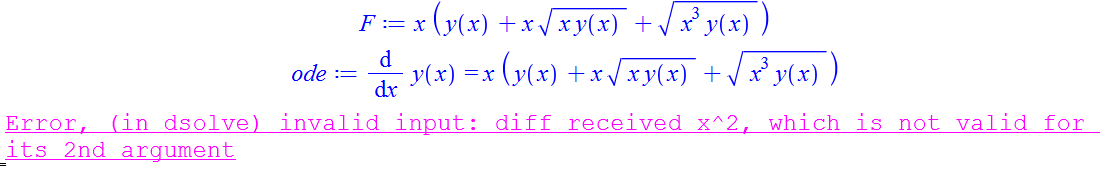I do not see where the input is invalid. I stared at it for 5 minutes.

Mathematica can solve this as follows

ode = y'[x] == x ( y[x] + x Sqrt[x y[x]] + Sqrt[x^3 y[x]]  )
DSolve[ode, y[x], x]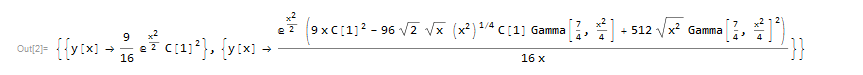Did I type something wrong in Maple?

Physics:-Version();
2018, June 12, 1:40 hours, MapleCloud version: 60

## how to tell Maple not to change expression when us...

I want z  to becomes abs(z) then later on, remove abs() and obtain z back in the same form it was.

I found that Maple changes z when I put it inside abs. Like this

expr:=(-y^2+1);
abs(expr);
op(1,%);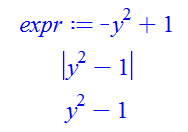So instead of 1-y^2, I end up now with  y^2-1. I want to keep the same expression I started with.

Mathematica does not do this:

expr = 1 - y^2
Abs[expr]
%[]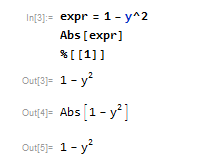Is there a way or option to tell Maple not change the expression when I put it inside abs()?

This complicates what I am trying to do in Maple.

thank you

## substitution of sub expressions. Is this an OK met...

What is the correct idiom in Maple to do subexpression replacement?

Suppose I want to replace each occurance of ln(anything) by ln(abs(anything)) in an expression.

Currently I call indets and then loop over each entry and use patmatch to do the replacement.

Is there a better method than what I doing now? Here is an example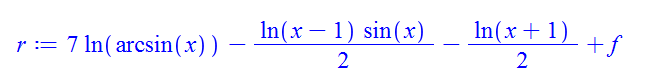restart;
expr := 7*ln(arcsin(x))-(1/2)*ln(x-1)*sin(x)-(1/2)*ln(x+1)+f;
lis:=indets(expr):
for z in lis do
a:='a';b:='b';c:='c';
if patmatch(z,a::anything*ln(b::anything)*c::anything,'la') then
map(z0->assign(z0),la);
expr:=subs(z = a*ln(abs(b))*c, expr);
fi;
od:
expr;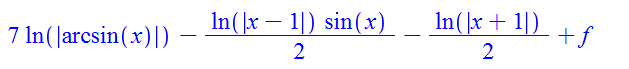I do not know if this will fails on some other cases yet.

## improving Latex output of Maple for expressions

by: Maple 2018

There are many questions that complain about Latex conversion in Maple.

I'd like to again request that Maplesoft improves Latex output of its expressions. If Maple can just fix how it generates fractions, that will good enough for now.

I am willing to send Maplesoft a personal check of the amount of one month salary for one of your developers to do this fix if you are willing to do it. It should not take more than one month to do this simple fix in your code. It might even take one day if someone knows the code.

The problem comes when there is a fraction in the expression. the Latex output instead of using proper latex code using "\frac{}{}", it instead uses "/" which makes the output terrible.

Another case, where Maple generate (expression)^{-1} instead of \frac{1}{expression}.

It can't be that hard to fix these 2 issues, which can go a long way towards making the latex generated by Maple much better. Here is an example

eq:=-(1/2)*1/y = (1/3)*x^3+z:
sol:=solve(eq,y);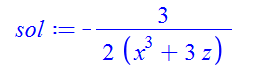latex(sol);
-3/2\, \left( {x}^{3}+3\,z \right) ^{-1}

Which renders as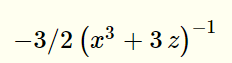Which is terrible. The screen output is much better.

Compare this to Mathematica

eq = -(1/2)*(1/y) == (1/3)*x^3 + z;
sol = y /. First@Solve[eq, y];
TeXForm[sol]

-\frac{3}{2 \left(x^3+3 z\right)}

Which renders in Latex as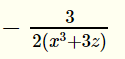If Maplesoft does not think Latex is improtant, then they are completely wrong. CAS support in Latex is very important. Ignoring Latex means you will lose customers who want good Latex support of the math output of Maple. After all, Math and Latex go togother. And Maple is supposed to be all about Mathematics.

Any chance of Maplesoft taking some time to fix these issues in Latex? Maple has not had any improvement in Latex for years and years. I keep buying Maple each year, and nothing changes in its Latex export.

thank you

## I can not export .eps file for density plot...

When I finished the following code, I can not export the .eps file for the densityplot

restart; t := 1; a := 0; a := 2; a := 0; a := 1; a := -1; a := 0; g := t*a+x*a+y*a+a; h := t*a+x*a+y*a+a; f := g^2+h^2+a; a := -(3*a^3+a*a^2+3*a*a^2-a*a^2+2*a*a*a)/(3*(a^2+a^2)); a := -(3*a^2*a+2*a*a*a-a^2*a+3*a^3+a*a^2)/(3*(a^2+a^2)); a := (3*(a^6+3*a^4*a^2+3*a^2*a^4+a^6))/(a*a-a*a)^2; u := (4*(2*a^2+a^2))/f-8*(g*a+h*a)^2/f^2; with(plots); plot3d(u, x = -20 .. 20, y = -20 .. 20, axes = frame, labels = ["x", "y", "z"], labeldirections = ["horizontal", "horizontal", "horizontal"], labelfont = ["TIMES", 16], style = patchnogrid); densityplot(u, x = -10 .. 10, y = -10 .. 10, axes = frame, labels = ["x", "y"], labeldirections = ["horizontal", "horizontal"], labelfont = ["TIMES", 16], colorstyle = HUE, style = patchnogrid); contourplot(u, x = -5 .. 5, y = -5 .. 5, labels = ["x", "y"], labeldirections = ["horizontal", "horizontal"], labelfont = ["TIMES", 16])

## Three argument signum...

Does anybody mind clarifying what the three argument signum means?

I have the expression

signum(0,R-sqrt(R^2+z^2),1)

in which z>0, R>0. I guess I know what signum means, however in this case according to the help it is considering signum of 0 and then in the help it is talking about some environment variable. I just dont quite understand...

Does it mean Maple has issues to clarify the sign of R-sqrt(R^2+z^2) ??

Thanks

﻿